### Home > CC2 > Chapter 8 > Lesson 8.3.4 > Problem8-107

8-107.
1. Evaluate each expression. Homework Help ✎

1.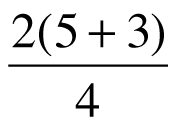2.(15 + 3) − 10 ÷ 2

3. 5− 2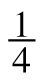+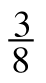4. 3 +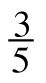·5. −2 + (−5 + 6)2

6.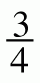·+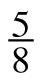· (−)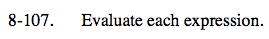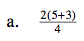Following the Order of Operations, first simplify the terms in parentheses, then the ones being multiplied or divided.
Lastly, combine any terms that are being added or subtracted.

4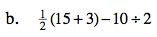See part (a).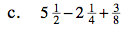You may find this expression easier to simplify by combining the whole numbers and the fractions separately.

$3\frac{5}{8}$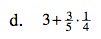See part (a).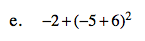First, simplify the terms in parentheses, then the terms with exponents, and lastly combing and numbers that are added or subtracted.

−1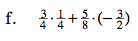See part (a).

$-\frac{3}{4}$